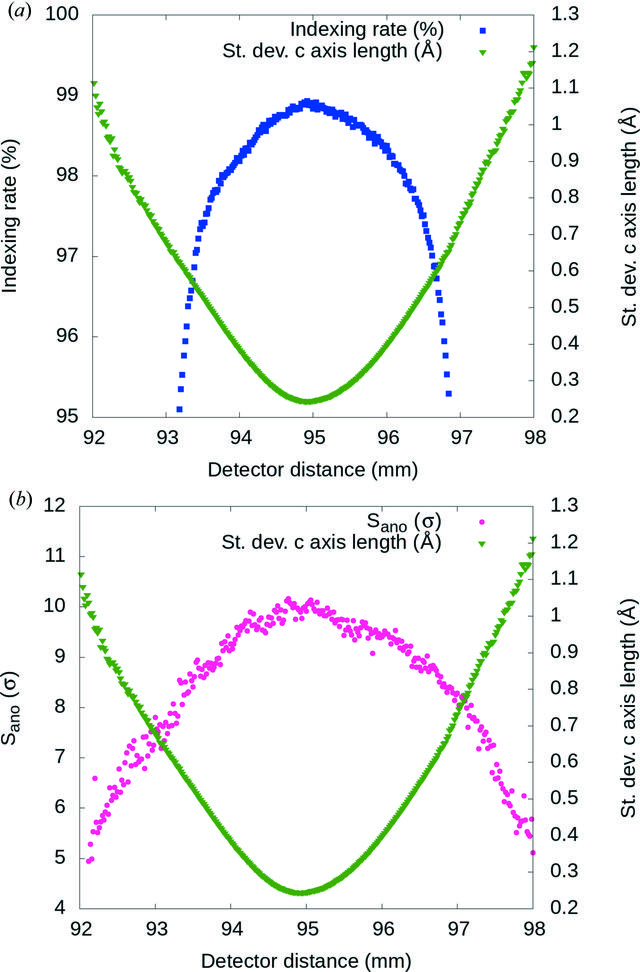disable zoom     view article Figure 2 Detector-distance optimization using thaumatin crystals. (a) The smallest standard deviation of the distribution of the unit-cell parameters (green triangles) indicates the optimal sample-to-detector distance 94.9 mm. The indexing rate (blue squares) is highest at the optimal detector distance. (b) Average peak height of the anomalous Fourier difference map (Sano) from thaumatin at 4.57 keV (pink circles) calculated at different detector distances. Sano is highest at the optimal detector distance which corresponds to the smallest standard deviation of c axis length (green triangles).

IUCrJ
ISSN: 2052-2525